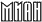Russian Academy of SciencesSteklov Mathematics Institutein cooperation withInstitute of Physics, Belgrade, Serbia andInternational Center for Mathematical Modelling in Physics,Engineering, Economy and Cognitive Sc., Vaxjo University, Sweden

The Third International Conference on p-Adic Mathematical Physics:
From Planck scale physics to complex systems to biology

 V. Anashin, Noncommutative algebraic dynamics (pdf file) V. Avetisov, A. Bikulov, S. Kozyrev, V. Osipov, A. Zubarev in cooperation with V. Ivanov and A. Chertovich, New results in applications of p-adic pseudo-differential equations to the protein dynamics (ppt file) Ya. Belopolskaya (joint with S. Albeverio), Stochastic processes in $Q_p$ associated (pdf file) T. Digernes, V. S. Varadarajan, D. Weisbart, Matrix Valued Schrödinger Operators on Local Fields (pdf file) B. Dragovich, A. Dragovich, Towards p-Adic Genomics (ppt file) K. Kamizono, $p$-adic Brownian Motion over $\mathbb Q_p$ (pdf file) S. V. Kozyrev, p-Adic numbers in genetics (pdf file) F. Murtagh, From Data to the p-Adic or Ultrametric Model (pdf file) F. Murtagh, Very high dimensional spaces are of simple structure (pdf file) V. Shelkovich (jointly with A. Yu. Khrennikov), p-Adic wavelets and their application to linear and nonlinear pseudo-differential evolutionary equations (pdf file) O. G. Smolyanov, N. N. Shamarov, Формулы Фейнмана и интегралы по траекториям в рамках p-адического анализа (in Russian, pdf file) M. Skopina (jointly with V. Shelkovich), p-Adic refinable functions and MRA-based wavelets (pdf file) R. G. Stepanov, Critical exponent $\nu$ in hierarchical models (pdf file) Z. Rakic (joint work with B. Dragovich), Path integrals for quadratic Lagrangians on real, p-adic, and adelic spaces (pdf file) V. S. Vladimirov, Boundary-value problems for open and closed p-adic strings theory (pdf file)© Steklov Mathematical Institute, 2007